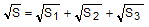Problem 142. Four Triangles, Incircle, Tangent and Parallel to Side, Areas.
 In the figure below, given a triangle ABC and the incircle O (inscribed circle), DE, FG, and HM are tangent to the incircle O and  parallel to AC, AB, and BC respectively. If S, S1, S2, S3 are the areas of triangles ABC, AHM, BDE, and CFG respectively, prove that. View or post a solution.See also: Typography of problem 142

Home | Geometry | Search | Wolfram Demonstrations Project | Problems | 141-150 | Email | by Antonio Gutierrez